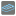• # Clearer explanation

## Question related to missionCipher dict

The wording for this problem is terrible. Thanks to the examples, I was able to understand what is expected and solve it. Here's my version of the subject.

The first part is quite clear, you need to encode your string in utf-8.
Then, you convert each character to its hexadecimal value. For 'hello', it gives '68656c6c6f'.
Then, you convert this hexadecimal value to decimals. This gives '448378203247'.

Then comes the tricky part.

You have to decompose your number in parts that are alternatively longest sequence of increasing digits and longest sequence of decreasing digits.

Example: with 153246, you would split it into 15 (increasing), 32 (decreasing) and 46 (increasing). This would be noted 15 / 32 / 46.

The next step is to associate a dictionary to each digit.
For our example, there are 6 digits so we have 6 digit/dictionary pairs: (1: dict1, 5: dict2) / (3: dict3, 2: dict4) / (4: dict5, 6: dict6).
Initially, all dictionaries are empty.

You create another empty dictionary to hold the final result. They call it A but I will call it dict0 instead, you will see why in an instant. The last thing we do is to give a number to each group of digit/dictionary pairs.

(1: dict1, 5: dict2) = group 0
(3: dict3, 2: dict4) = group 1
(4: dict5, 6: dict6) = group 2

Now, what you need to do is to put all the digit/dictionary pairs of group 0 into dict0, all the digit/dictionary pairs of group 1 into dict1, etc.

group 0 into dict0 -> dict0 = {1: dict1, 5: dict2}
group 1 into dict1 -> dict0 = {1: {3: dict3, 2: dict4}, 5: dict2}
group 2 into dict2 -> dict0 = {1: {3: dict3, 2: dict4}, 5: {4: dict5, 6: dict6}}

Final result: {1: {3: {}, 2: {}}, 5: {4: {}, 6: {}}}23